Courses

# Physics Test 6 - Modern Physics

## 30 Questions MCQ Test Mock Test Series for JEE Main & Advanced 2021 | Physics Test 6 - Modern Physics

Description
This mock test of Physics Test 6 - Modern Physics for JEE helps you for every JEE entrance exam. This contains 30 Multiple Choice Questions for JEE Physics Test 6 - Modern Physics (mcq) to study with solutions a complete question bank. The solved questions answers in this Physics Test 6 - Modern Physics quiz give you a good mix of easy questions and tough questions. JEE students definitely take this Physics Test 6 - Modern Physics exercise for a better result in the exam. You can find other Physics Test 6 - Modern Physics extra questions, long questions & short questions for JEE on EduRev as well by searching above.
QUESTION: 1

### An electromagnetic waves going through vacuum is described by,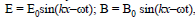Then

Solution:

The relation between E0 and B0 is given by E0/B0 = c ..(1)
Here, c = speed of the electromagnetic wave
The relation between ω (the angular frequency) and k (wave number):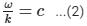Therefore, from (1) and (2), we get: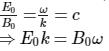QUESTION: 2

### Because of the space charge in a diode valve

Solution: Electrons from the cathode surface of the diode valve are emitted into this negative field and their velocities will vary with different electrons. The negative field around the cathode causes the electrons to slow as they are repelled by the negative charge. the space charge in a diode valve the plate current decreases
QUESTION: 3

### A point source of light is used in a photoelectric effect. If the source is removed farther from the emitting metal, the stopping potential

Solution:

As source is moved away, intensity decreases but frequency remains same.
∴ No effect on the stopping potential.

QUESTION: 4

Moseley’s law for characteristic X-ray is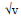=a(z-b).In this

Solution:
QUESTION: 5
The X-ray beam coming from an X-ray tube
Solution:
QUESTION: 6

Cutoff wavelength of X-rays coming from a Coolidge tube depends on the

Solution:

Cutoff wavelength (λmin) is given by

λmin = hceV

Here,

h = Planck's constant

c = Speed of light

V = Accelerating voltage

e = Charge of electron

Clearly, a cutoff wavelength depends on accelerating voltage. It does not depend on the target material, separation between the target and the temperature of the filament.

Answer: Accelerating voltage.

QUESTION: 7

Two identical capacitors A and B are charged to the same potential V and are connected in two circuits at t = 0 as shown in figure. The charges on the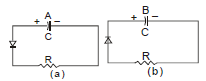capacitors at a time = CR are, respectively

Solution:
QUESTION: 8
In a transistor
Solution:
QUESTION: 9

A semiconducting device is connected in a series circuit with a battery and a resistance. A current is found to pass through the circuit. If the polarity of the battery is reversed, the current drops to almost zero. The device may be

Solution:
QUESTION: 10

In a radioactive decay, neither the atomic number nor the mass number changes. Which of the following particles is emitted in the decay ?

Solution:
QUESTION: 11

Ten grams of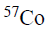kept in an open containg beta-decays with a half-life of 270 days. The weigth of the material inside the container after 540 days will be very nearly

Solution:

57Co is undergoing beta decay, i.e. electron is being produced. But an electron has very less mass (9.11 x10^-31 kg) as compared to the Co atom. Therefore, after 570 days, even though the atoms undergo large beta decay, the weight of the material in the container will be nearly 10 g.

QUESTION: 12

A free neutron decays to a proton but a free proton does not decay to a neutron. This is because

Solution:
QUESTION: 13

If elements with principle quantum number n>4 were not allowed in nature, the number of possible elements would be

Solution:

N=1 present K-shell and the number of elements having K shell=2 N=2 present L shell and the number of element having L shell=8 N=3 present N shell and the number of elements having N shell = 18 N=4 present N shell and the number of elements having N shell = 32 Total number = 2+8+18+32=60

QUESTION: 14

Consider the spectral line resulting from the transition n =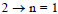in the atoms and ions given below. The shortest wave length is produced by

Solution: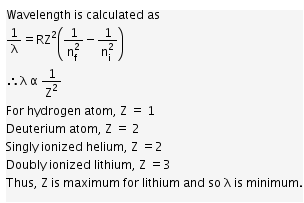QUESTION: 15
The equation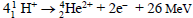represents
Solution:
QUESTION: 16

The probability of electrons to be found in the conduction band of an intrinsic semiconductor at a finite temperature

Solution:
QUESTION: 17

The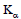X-ray emission line of tungsten occurs at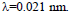The energy difference between K and L levels in this atom is about

Solution:
QUESTION: 18

The electrical conductivity of a semiconductor increases.
when em radiation of wavelength shorter than 2480 nm is incident on it. The band gap (in eV) for the semiconductor is

Solution:
QUESTION: 19

The circuit shown in the figure contains tow diodes each with a forward resistance of 50 ohms and with infinite backward resistance. If the battery voltage is 6V, the current through the 100 ohm resistance (in Amperes) is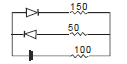Solution:
QUESTION: 20

Which of the following statement is not ture ?

Solution:
QUESTION: 21

Binding energy per nucleon vs. mass number curve for nuclei is shown in the figure W,X,Y and Z are four nuclei indicated on the curve. The process that would release energy is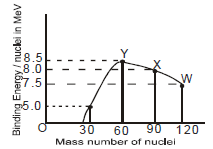Solution:
QUESTION: 22

The potential difference applied to an X-ray tube is 5kV and the current through it is 3.2 mA. Then the number of electrons striking the target per second is

Solution:

n=Ite

=3.2x10^−3x11.6x10^−19

=2x10^16

QUESTION: 23

A Hydrogen atom and a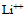ion are in the second excited state.If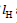and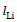are their respectively electronic angular momenta, and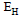and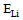their respectively energies, then

Solution:
QUESTION: 24

In a photoelectric experiment anode potenial is plotted against plate current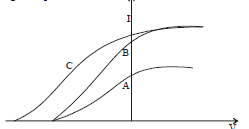Solution:
QUESTION: 25

Direction : Read the following question and choose
A. If both assertion and reason are true and reason is the correct explantion of assertion
B. If both assertion and reason are true but reason is not the correct explanation of assertion
C. If assertion is true but reason is false
D. If assertion is false but reason is true
Assertion : Nuclear density is almost same for all nuclei
Reason: Size of nucleus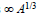Solution:
QUESTION: 26

Direction : Read the following question and choose
A. If both assertion and reason are true and reason is the correct explantion of assertion
B. If both assertion and reason are true but reason is not the correct explanation of assertion
C. If assertion is true but reason is false
D. If assertion is false but reason is true
Assertion: A free neutron is not a stable particle
Reason: It decays spontaneously into a proton, an electron and an antineutrino

Solution:
QUESTION: 27
Direction : Read the following question and choose
A. If both assertion and reason are true and reason is the correct explantion of assertion
B. If both assertion and reason are true but reason is not the correct explanation of assertion
C. If assertion is true but reason is false
D. If assertion is false but reason is true
Assertion: In outermost stationary orbit, energy of electron is least negative
Reason: In such an orbit, electron is at maximum distance from the nucleus
Solution:
QUESTION: 28
Direction : Read the following question and choose
A. If both assertion and reason are true and reason is the correct explantion of assertion
B. If both assertion and reason are true but reason is not the correct explanation of assertion
C. If assertion is true but reason is false
D. If assertion is false but reason is true
Assertion : Nuclear forces are non-central forces
Reason : Nuclear forces do not act neccessarily along the line joining their centers
Solution:
QUESTION: 29
Direction : Read the following question and choose
A. If both assertion and reason are true and reason
is the correct explantion of assertion
B. If both assertion and reason are true but reason is
not the correct explanation of assertion
C. If assertion is true but reason is false
D. If assertion is false but reason is true
Assertion : X-rays travel with the speed of light
Reason : X-rays are electromagnetic rays
Solution:
QUESTION: 30
Direction : Read the following question and choose
A. If both assertion and reason are true and reason is the correct explantion of assertion
B. If both assertion and reason are true but reason is not the correct explanation of assertion
C. If assertion is true but reason is false
D. If assertion is false but reason is true
Assertion : The conductivity of intrinsic semiconductors at 0 K is zero
Reason: At 0 K no energy is available. No covalent bond can be broken. No free electron is available for conduction

Solution: